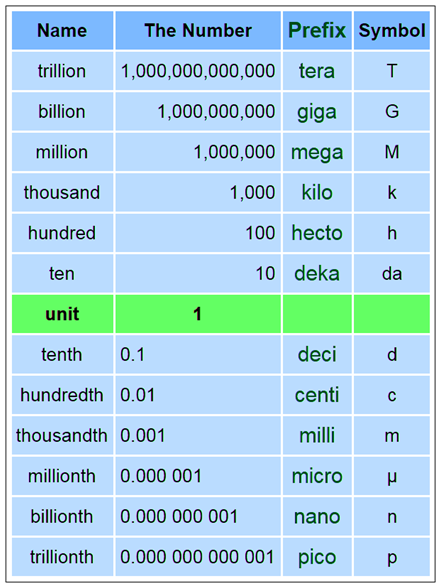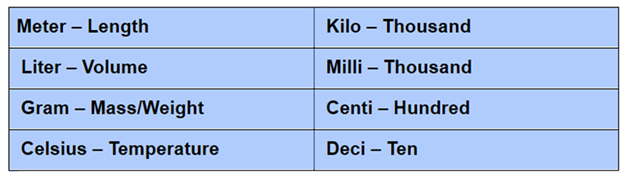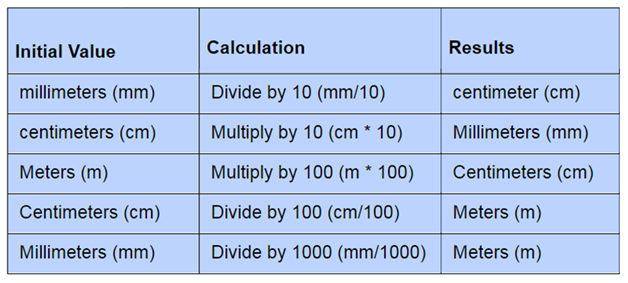# Metric System

Metric System is basically a system used for measuring distance, length, volume, weight and temperature. It is based on three basic units with which we can measure almost everything in the world

1. M- meter, used to measure the length
2. Kg- kilogram, used to measure the mass
3. S- second, used to measure time

The metric system was originated in the year 1799. Over the years, this system has been extended to incorporate many units. Although a number of variants of this system were discovered in the late 19th and early 20th centuries, the term – metric system is used as another word for SI or the International System of Units.

## SI Units Metric System

The SI Units (standard International System of Units) give proper definitions for meter, kilogram and the second. It also specifies and defines four different additional units :

Kelvin- temperature

Ampere – Electric Current

Candela- luminous Intensity

Mole – Material Quantity

## Metric Number Prefixes

In order to remember the proper movement of units, arrange the prefixes from the largest to the smallest.

 Kilo hecto deca Unit deci centi milli 103 102 101 100 10-1 10-2 10–3

Thus, the conversion from one unit to the other unit is done by multiplying or dividing the powers of 10.

Now, let us discuss some of the units for length, weight, volume, and time.

Length:

The most common units used to measure the length are as follows:

 Kilometer (km) Hectometer (hm) Decameter (dam) Meter (m) Decimeter (dm) Centimeter (cm) Millimeter (mm) 1000 100 10 1 1/10 1/100 1/1000

Also, check: Centimeter

Volume or Capacity

The most common units used to measure the capacity or volume of any object are as follows:

 Kiloliter (kl) Hectoliter (hl) Decaliter(dal) Liter (l) Deciliter (dl) Centiliter (cl) Milliliter(ml) 1000 100 10 1 1/10 1/100 1/1000

Weight

The most common units used to measure the weight of any object are as follows:

 Kilogram (kg) Hectogram (hg) Decagram (dag) Gram (g) Decigram (dg) Centigram  (cg) Milligram (mg) 1000 100 10 1 1/10 1/100 1/1000

Time

To calculate the time, the base unit is second. Now, let us discuss some other units of time.

1 minute = 60 seconds

1 hour = 60 minutes

1 day = 24 hours

1 week = 7 days

1 month = 30 or 31 days (Note: February has 28 days, but in leap year February has 29 days)

1 year = 12 months (or)

1 year = 365 days (But, in leap year, 1 year = 366 days)

Whether you want to measure very small or very big things, You can go for metric number prefixes—for example- kilo and milli.There are some common units that are based on the kilogram, meter and second.

1. Area = Square Meter (Area= length by length) Therefore the basic unit of area is equal to meters by meters = m2 (square meters).
1. Volume = Cubic Meter. Therefore, the basic unit of volume = m3 (cubic meters).
1. Liter = one-thousandth of a cubic meter(1 m3 = 1,000 Liters) Therefore, 1 Liter = 1/1000 m3
1. Time = Hour (1 hour=60 minutes, 1 minutes=60 seconds) Therefore,1 hour= 60×60= 3600 seconds
1. Day = (1 day= 24 hours) Therefore, 1 day= 24 x 60 x 60 = 86400 seconds

Important Things to Remember### Metric System Conversion

We can follow different methods for metric conversions. The below metric conversion chart shows the conversion of Metric Units to Metric Units for Length.## Conversion Ratio

In the measurement of units, the metric conversion ratio is a ratio, which is equal to 1. It is also known as unit factors. It is used to convert the units between the same system, as well as the conversion between the metric and the English systems. It is based on the concept of equivalent values. Similarly, a conversion factor is defined as a number that is used to convert one unit to another unit by dividing or multiplying to get the equivalent values.

For example, to convert 5 meters to centimetres, a conversion factor is required.

We know that 1 meter = 100 centimetres

Thus, the conversion factor is “multiplying by 100”.

(i.e.,) 5 m = 5 x 100 cm

5 m = 500 cm

Therefore, the conversion from 5 m to cm is 500 cm.

In terms of conversion ratio, it can be written as:

5m / 500 cm = 1

### Metric System Examples

The following examples explain the conversion from one unit to the other unit.

Example 1:

Convert 356 centimetres to meters.

Solution:

We know that, 1 cm = 0.01 m

Thus, 356 cm = 356 x 0.01

356 cm = 3.56 m.

Therefore, 356 cm is equivalent to 3.56 meters.

Example 2:

Convert 4000 grams to kilograms.

Solution:

We know that, 1 gram = 0.001 kilogram

Thus, 4000 g = 4000 x 0.001

4000 g = 4 kilogram

Therefore, 4000 grams is equivalent to 4 kilogram

Example 3:

Convert 50 kilolitres to liters.

Solution:

We know that 1 kilolitre = 1000 liters

Thus, 50 kilolitres = 50 x 1000 litres

50 kilolitres = 50000 litres

Therefore, 50 kilolitres are equivalent to 50000 liters.

## Frequently Asked Questions on Metric Systems

Q1

### What is meant by the metric system?

The metric system is defined as the system of measurements to calculate the mass, distance, and volume of any object. We generally use the metric system to measure smaller or larger quantities.

Q2

### What are the base units for length, weight, and volume in a metric system?

In the metric system, the base units for length, weight, and volume are meters, grams, and liters respectively.

Q3

### Mention the US standard units for length, weight, and volume.

In US customary systems, the units used are:
Distance or length in miles, yards, feet, inches
Mass or weight in pounds, tons, ounces
Capacity or volume in cups, gallons or quarts, pints, fluid ounces.

Q4

### What is meant by metric number prefixes?

The metric number prefix is defined as a unit prefix, which precedes a base unit to indicate the multiple or the submultiple of a unit. Some of the metric prefixes are:
Kilo (1000 or 103)
Hecto (100 or 102)
Deca (10 or 101)
Base unit (1)
Deci (1/10 or 10-1)
Centi (1/100 or 10-2)
Milli (1/1000 or 10-3)

Q5

### Convert 80 grams to milligrams.

We know that 1 gram = 1000 milligrams
Therefore, 80 grams = 80 x 1000 milligrams
80 grams = 80000 milligrams
Thus, 800 grams is equivalent to 80000 milligrams.

Quiz on Metric system